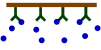### BIA-Pagesback
Association
back
back

# Association

The association is the first phase in a biomolecular interaction experiment. Binding occurs when analyte and ligand collide due to diffusion, and when the collision has the correct orientation and enough energy. The rate of association (number of binding events per unit of time) equals:

Once binding has occurred, the ligand and analyte remain bound together for a random amount of time. The rate of dissociation (number of dissociation events per unit of time) equals:

The probability of dissociation is the same every instant of time. The analyte does not "know" how long it has been bound to the ligand. After dissociation, the ligand and analyte are the same as they were before binding. If either analyte or ligand is chemically modified, then the binding does not follow the law of mass action (1,2).

## Kinetic equations

The reaction between immobilized ligand (L) and an analyte (A) can be assumed to follow a pseudo first order kinetics (3, 4, 6). During the association phase, the complex [LA] increases as a function of time according to:

The association rate constant ka describes the rate of complex formation, i.e. the number of LA complexes formed per second in a one molar solution of L and A. The units of ka are M-1s-1 and are typically between 1.103 and 1.107 in biological systems.

The dissociation rate constant kd describes the stability of the complex, i.e. the fraction of complexes that decays per second. The unit of kd is s-1 and is typically between 1.10-1 and 1.10-6 in biological systems. A kd of 1.10-2 s-1 = 0.01 s-1. This means that 1 percent of the complexes decay per second (5).

## Integrated rate equations

Fitting the kinetic equation is not an easy task. One way is the transformation of the kinetic equation to a so called integrated rate equation, which can be resolved by computer programs.